# Learning R: Permutations and Combinations with base R

June 3, 2019
By

Want to share your content on R-bloggers? click here if you have a blog, or here if you don't.The area of combinatorics, the art of systematic counting, is dreaded territory for many people so let us bring some light into the matter: in this post we will explain the difference between permutations and combinations, with and without repetitions, will calculate the number of possibilities and present efficient R code to enumerate all of them, so read on…

The cases we cover in this post:

• Permutations with repetitions
• Combinations without repetitions
• Permutations (of all elements) without repetitions

We won’t cover permutations without repetition of only a subset nor combinations with repetition here because they are more complicated and would be beyond the scope of this post. We will perhaps cover those in a later post.

In all cases, you can imagine somebody drawing elements from a set and the different ways to do so. Permutation implies that the order does matter, with combinations it does not (e.g. in a lottery it normally does not matter in which order the numbers are drawn). Without repetition simply means that when one has drawn an element it cannot be drawn again, so with repetition implies that it is replaced and can be drawn again.

Let us start with permutations with repetitions: as an example take a combination lock (should be permutation lock really!) where you have three positions with the numbers zero to nine each. We use the expand.grid() function for enumerating all possibilities:

P_wi <- expand.grid(rep(list(0:9), 3))
##   Var1 Var2 Var3
## 1    0    0    0
## 2    1    0    0
## 3    2    0    0
## 4    3    0    0
## 5    4    0    0
## 6    5    0    0

tail(P_wi)
##      Var1 Var2 Var3
## 995     4    9    9
## 996     5    9    9
## 997     6    9    9
## 998     7    9    9
## 999     8    9    9
## 1000    9    9    9


The formula for calculating the number of permutations is simple for obvious reasons (is the number of elements to choose from,is the number of actually chosen elements):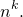In R:

10^3
##  1000

nrow(P_wi)
##  1000


The next is combinations without repetitions: the classic example is a lottery where six out of 49 balls are chosen. We use the combn() function for finding all possibilities:

C_wo <- combn(1:49, 6)
C_wo[ , 1:10]
##      [,1] [,2] [,3] [,4] [,5] [,6] [,7] [,8] [,9] [,10]
## [1,]    1    1    1    1    1    1    1    1    1     1
## [2,]    2    2    2    2    2    2    2    2    2     2
## [3,]    3    3    3    3    3    3    3    3    3     3
## [4,]    4    4    4    4    4    4    4    4    4     4
## [5,]    5    5    5    5    5    5    5    5    5     5
## [6,]    6    7    8    9   10   11   12   13   14    15


To calculate the number of combinations the binomial coefficient is used: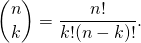To give you some intuition consider the above example: you have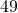possibilities for choosing the first ball,for the second,for the third and so on up to the sixth ball. So that gives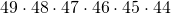. When you think about it this is the same asbecause all the coefficients smaller than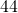can be eliminated by reducing the fraction! Now, there arepossible positions for the first ball that is drawn,for the second… and so on and because the order doesn’t matter we have to divide by, which gives the binomial coefficient. In R we use the choose() function to calculate it:

choose(49, 6)
##  13983816

ncol(C_wo)
##  13983816


So, you see that the probability of winning the lottery are about the same, no matter whether you play it… or notOur last case is permutations (of all elements) without repetitions which is also the most demanding one because there is no readily available function in base R. So, we have to write our own:

perm <- function(v) {
n <- length(v)
if (n == 1) v
else {
X <- NULL
for (i in 1:n) X <- rbind(X, cbind(v[i], perm(v[-i])))
X
}
}


As you can see it is a recursive function, to understand recursion read my post: To understand Recursion you have to understand Recursion…. What makes matters a little bit more complicated is that the recursive call is within a for loop.

The idea is to fix one element after the other [for (i in 1:n) and cbind(v[i], ...)] and permute the remaining elements [perm(v[-i])] down to the base case when only one element remains [if (n == 1) v], which cannot be permuted any further. Collect all sets on the respective higher level [X <- (rbind(X, ...)] and return the whole matrix X.

Let us see this in action, as an example we’ll see how many different ways there are of four runners reaching the finishing line:

P_wo <- perm(1:4)
P_wo
##       [,1] [,2] [,3] [,4]
##  [1,]    1    2    3    4
##  [2,]    1    2    4    3
##  [3,]    1    3    2    4
##  [4,]    1    3    4    2
##  [5,]    1    4    2    3
##  [6,]    1    4    3    2
##  [7,]    2    1    3    4
##  [8,]    2    1    4    3
##  [9,]    2    3    1    4
## [10,]    2    3    4    1
## [11,]    2    4    1    3
## [12,]    2    4    3    1
## [13,]    3    1    2    4
## [14,]    3    1    4    2
## [15,]    3    2    1    4
## [16,]    3    2    4    1
## [17,]    3    4    1    2
## [18,]    3    4    2    1
## [19,]    4    1    2    3
## [20,]    4    1    3    2
## [21,]    4    2    1    3
## [22,]    4    2    3    1
## [23,]    4    3    1    2
## [24,]    4    3    2    1


After this rather complicated function the calculation of the number of ways is simple, it is just the factorial function (it should again be obvious why):In R we have the factorial() function:

factorial(4)
##  24

nrow(P_wo)
##  24


As you will see when solving real world problems with R the above functions often come in handy, so you should add them to your ever growing tool set – have fun and stay tuned!

R-bloggers.com offers daily e-mail updates about R news and tutorials about learning R and many other topics. Click here if you're looking to post or find an R/data-science job.
Want to share your content on R-bloggers? click here if you have a blog, or here if you don't.